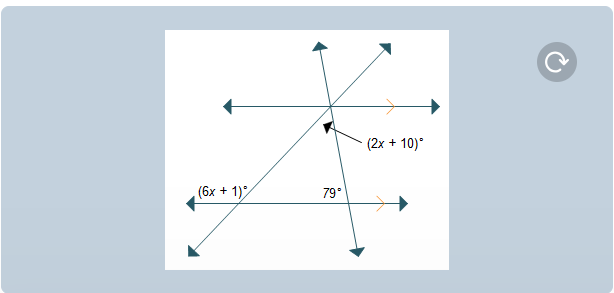# What is the value of x? x = 2.25 x = 11.25 x = 13 x = 22?

What is the value of x? x = 2.25 x = 11.25 x = 13 x = 22?